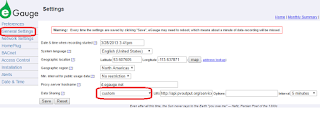# Updating pvoutput.org with solar output from eGauge

If you have solar panels, logging your generated data to pvoutput.org lets you compare your system performance with others in your area.Solar output graph from pvoutput.org

I have an eGauge energy meter measuring solar generation as well as consumption.

Update 28 May 2016 As Sandeen points out in the comments, you can have eGauge push the data to pvoutput directly (introduced in April 2016). Under "General Settings", set Data Sharing mode to Custom and enter the pvoutput api url in the URI : http://api.pvoutput.org/service/r2/egaugepost.jsp?sid=your-sid&key=your-key&tz=Canada/MountainSince pvoutput.org does not directly support pulling data from eGauges, I wrote a python script to pull the data from the eGauge and post it to pvoutput.org once a week.

```import datetimeimport timeimport pandas as pdimport urllib2import xmltodictimport requestsfrom tzlocal import get_localzonedef egaugeSolarProduction(url, numberOfDays, endingOnDate):    """ Fetches Solar+ generation data from an eGauge for a given number of days.    :param url: address of the eGauge    :param numberOfDays: how many days to return data for.    :param endingOnDate: last date to return data for.    :return: pandas series with datetime index and Wh (watt-hour) values for the given day.    """    data = []    timestamps = []        localTimeZone = get_localzone()        endOfDay = datetime.datetime(endingOnDate.year, endingOnDate.month, endingOnDate.day, 0, 0, 0) + datetime.timedelta(days=1)    endOfDay = endOfDay.replace(tzinfo = localTimeZone)        command = url + "/cgi-bin/egauge-show?d&n=" + str(numberOfDays + 1) + "&Z=MDT&f=" + str(hex(int(time.mktime(endOfDay.astimezone(pytz.UTC).timetuple()))))    response = urllib2.urlopen(command) # does a GET since no parameters were passed     eGaugeData = response.read()    response.close()    result = xmltodict.parse(eGaugeData)       timeDelta = int(result['group']['data']["@time_delta"])    startTime = time.gmtime(int(result['group']['data']["@time_stamp"], 16))    generatedColumnNumber = -1    currentColumnNumber = 0    for meta in result['group']['data']['cname']:        if meta['#text'] == "Solar+":            generatedColumnNumber = currentColumnNumber        currentColumnNumber += 1              timeStamp = localTimeZone.fromutc(datetime.datetime.fromtimestamp(time.mktime(startTime)))    powerRegister = -1    for d in result['group']['data']['r']:        if powerRegister > 0:            energyInWattSeconds = powerRegister - int(d['c'][generatedColumnNumber])            data.append(energyInWattSeconds / 3600)            timestamp = (timeStamp - datetime.timedelta(seconds=timeDelta)).replace(tzinfo=None)            timestamps.append((timeStamp + datetime.timedelta(seconds=timeDelta)).replace(tzinfo=None))        powerRegister = int(d['c'][generatedColumnNumber])        timeStamp = timeStamp - datetime.timedelta(seconds=timeDelta)            return pd.Series(data, index=timestamps).sort_index()   def uploadToPvoutput(apikey, sid, date, generated):      """ Uploads solar generation data to pvoutout.    :param apikey: pvoutput.org api key (see http://pvoutput.org/account.jsp)    :param sid: ovoutput.org system id (see http://pvoutput.org/account.jsp)    :param date: date solar energy was generated    :param generated: solar generation for given date in Wh (Watt-hours)    """    r = requests.post("http://pvoutput.org/service/r2/addoutput.jsp", headers={'X-Pvoutput-ApiKey': apiKey, 'X-Pvoutput-SystemId': sid}, data={'d': date.strftime("%Y%m%d"), 'g': generated});    if r.status_code != requests.codes.ok:        print("There was a problem updating pvoutput.org: code " + r_status_code)# Put your api key and system id belowapiKey = "b1203c7c733bb12349888f789234466461781111"systemId = 42481numberOfDaysToUpdate = 7# Get all solar generation data for the last 7 days (not including today)yesterday = datetime.datetime.now() - datetime.timedelta(days=1)data = egaugeSolarProduction("http://egauge1234.egaug.es", numberOfDaysToUpdate, yesterday)for day in data.index:    print("Updating pvoutput.org: date " + day.strftime("%d-%m-%Y") + " " + "%0.2f Wh" %(data[day]))    uploadToPvoutput(apiKey,systemId, day, data[day])    print("Total last %d days: %0.2f kWh generated" %(numberOfDaysToUpdate, data.sum() / float(1000)))
```

No, that is not my real api key in the above codeI run this script once a week, and it prints this:
```Updating pvoutput.org: date 31-12-2015 85.00 WhUpdating pvoutput.org: date 01-01-2016 225.00 WhUpdating pvoutput.org: date 02-01-2016 520.00 WhUpdating pvoutput.org: date 03-01-2016 1503.00 WhUpdating pvoutput.org: date 04-01-2016 4005.00 WhUpdating pvoutput.org: date 05-01-2016 5912.00 WhUpdating pvoutput.org: date 06-01-2016 2761.00 WhTotal last 7 days: 15.01 kWh generated
```